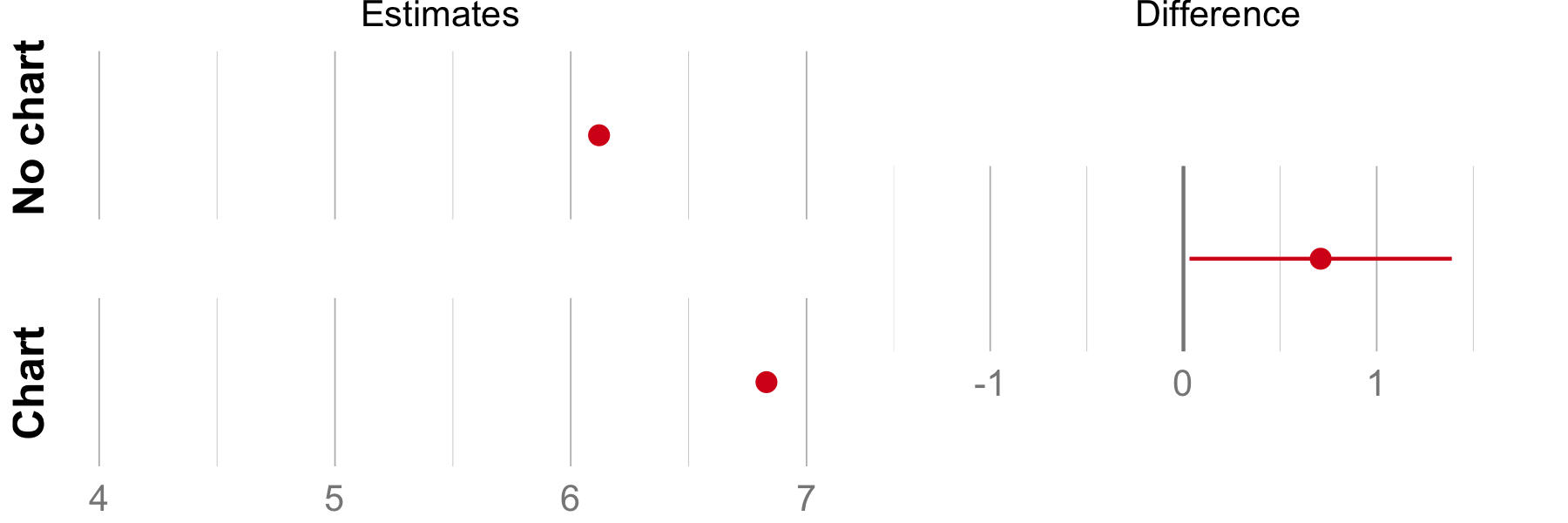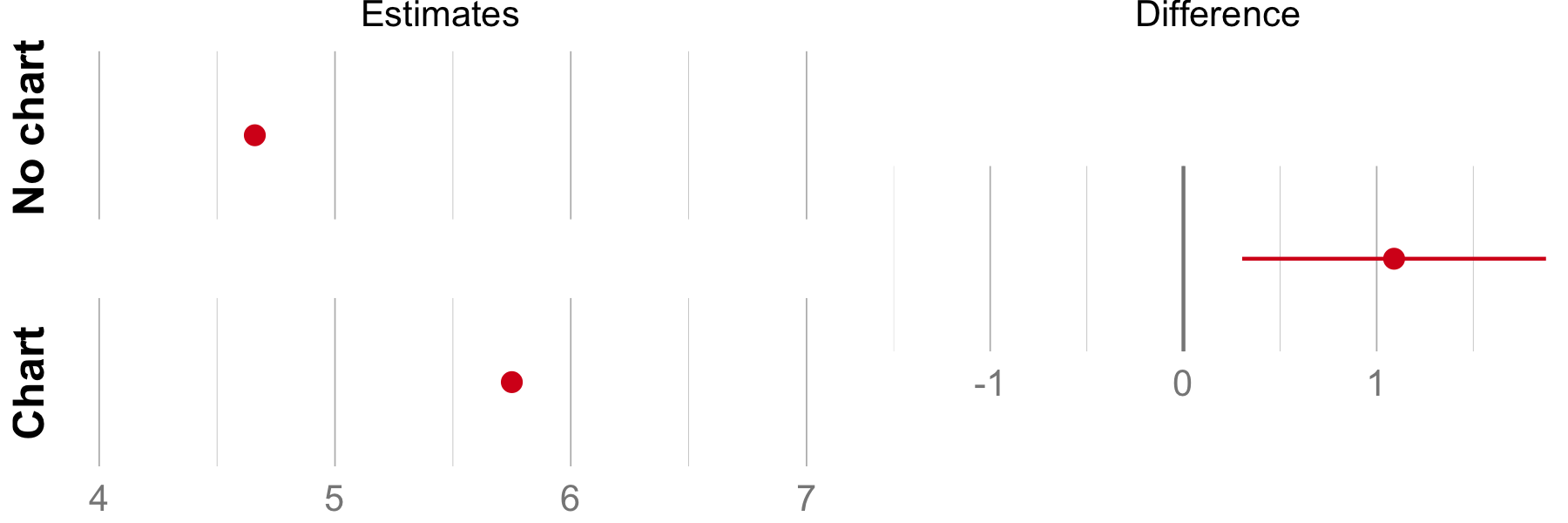``````############################################################################
``````

# Various reanalyses of Tal and Wansink (2014)

From the information reported in their paper.

2017 Pierre Dragicevic, Yvonne Jansen Created March 2017, cleaned up August 2017

``````###########################################################################

rm(list = ls())

source("CI.helpers.R")
source("misc.helpers.R")
source("plotting functions.R")

knitr::opts_chunk\$set(fig.width=8, fig.height=3, fig.path="figures/", fig.retina = 2, dpi = 150, fig.align='center')
########################################################################
``````

## BwS experiment 2 – Interval estimates for retention question

``````########################################################################
``````

On p.3 we report that the 95% CI for the difference between the proportions of correct answers in the retention question was [-17%, 30%]. This section explains how we obtained this result. From the BwS paper: “Participants for Study 2 (N=56, 53% male, mean age 19.08) were recruited from the participant pool of a behavioral lab in a large Northeastern University.”

``````N <- 56
``````

Let us first assume equal sample size across the two conditions:

``````N_nograph <- N/2
N_graph <- N/2
``````

From the paper: “The percentage of participants who correctly reported reduction in illness (within 3% error) was not greater for the graphs condition, with 78.95% (vs 70.27%) reporting correctly in the control (graphs) condition (p > .2).”

``````pcorrect_nograph <- 78.95
ncorrect_nograph <- round(N_nograph * pcorrect_nograph / 100)
pcorrect_graph <- 70.27
ncorrect_graph <- round(N_graph * pcorrect_graph / 100)
``````

Test whether we obtain the same rounded percents

``````cat('Percent correct for no graph: ', round(ncorrect_nograph / N_nograph * 100, 2),'\n', sep="")
``````
``````## Percent correct for no graph: 78.57
``````
``````cat('Percent correct for graph: ', round(ncorrect_graph / N_graph * 100, 2),'\n\n', sep="")
``````
``````## Percent correct for graph: 71.43
``````

We don't. Try to infer the most likely sample size for each condition using the function retrieveCounts (see misc.helpers.R)

``````print(retrieveCounts(pcorrect_nograph, N, 0.1), row.names = F)
``````
``````##   n  N        p       error
##  15 19 78.94737 0.002631579
##  30 38 78.94737 0.002631579
``````
``````print(retrieveCounts(pcorrect_graph, N, 0.1), row.names = F)
``````
``````##   n  N        p        error
##  26 37 70.27027 0.0002702703
##  33 47 70.21277 0.0572340426
``````

They're 19 and 37, summing up to N=56.

``````N_nograph <- 19
N_graph <- 37
``````

Recompute n correct

``````ncorrect_nograph <- round(N_nograph * pcorrect_nograph / 100)
ncorrect_graph <- round(N_graph * pcorrect_graph / 100)
``````

Verify again the percents

``````cat('Percent correct for no graph: ', round(ncorrect_nograph / N_nograph * 100, 2),'\n', sep="")
``````
``````## Percent correct for no graph: 78.95
``````
``````cat('Percent correct for graph: ', round(ncorrect_graph / N_graph * 100, 2),'\n\n', sep="")
``````
``````## Percent correct for graph: 70.27
``````

They're correct. Now compute the CI

``````format_ci(diffpropCI(ncorrect_nograph, N_nograph, ncorrect_graph, N_graph), percent = T)
``````
``````##  "8.7%, CI [-17%, 30%]"
``````
``````########################################################################
``````

## BwS experiment 1 – Interval estimates for perceived effectiveness

``````########################################################################
``````

These are the values reported in the BwS paper

``````bws_nochart <- 6.12
bws_chart <- 6.83
bws_p <- 0.04
``````

We put these results into a dataframe for plotting using the same chart type as for own results. Without the raw data from this experiment, we cannot compute confidence intervals around the mean estimates from the BwS paper.

``````eff_df <- NULL
eff_df <- add.ci.to.df(c(bws_nochart, bws_nochart, bws_nochart), "no chart", "BwS", eff_df)
eff_df <- add.ci.to.df(c(bws_chart, bws_chart, bws_chart), "chart", "BwS", eff_df)
``````

For the difference between the two conditions, we can use the p-value indicated in their article to compute the confidence interval around the difference. We use the method by D.G.Altman, J.M.Bland: How to obtain the confidence interval from a p value. BMJ, 343:d2090, 2011. https://doi.org/10.1136/bmj.d2090

``````eff_diff_df <- NULL
eff_diff_df <- add.ci.to.df(ci_from_p(bws_chart - bws_nochart, bws_p), " ", "BwS", eff_diff_df)
``````

Now we plot the results

``````eff.cis <- perceived.effectiveness.1.ciplot(eff_df, eff_diff_df)
drawHorizontalEfficiency(eff.cis, "Estimates", "Difference")
````````````########################################################################
``````

## BwS experiment 1 – Interval estimates for belief in efficacy (binary)

``````########################################################################
``````

On p.5 we report the 95% CIs for the proportion of people who believed the medication really reduced illness, for each of the two conditions and for the difference. This section explains how we derived the CIs. Total sample size for this experiment

``````N <- 61
``````

Percentage of people who replied yes

``````p_yes_nograph <- 67.74
p_yes_graph <- 96.55
``````

As before, find the most likely sample sizes per condition

``````print(retrieveCounts(p_yes_nograph, N, 0.1), row.names = F)
``````
``````##   n  N        p       error
##  21 31 67.74194 0.001935484
##  40 59 67.79661 0.056610169
##  23 34 67.64706 0.092941176
``````
``````print(retrieveCounts(p_yes_graph, N, 0.1), row.names = F)
``````
``````##   n  N        p       error
##  28 29 96.55172 0.001724138
##  56 58 96.55172 0.001724138
##  55 57 96.49123 0.058771930
##  57 59 96.61017 0.060169492
``````

They're 31 and 29, summing up to N=60.

``````N_nograph <- 31
N_graph <- 29
N <- N_nograph + N_graph
``````

Recompute the counts

``````yes_nograph <- round(N_nograph * p_yes_nograph / 100)
yes_graph <- round(N_graph * p_yes_graph / 100)
``````

Verify the percentages

``````cat('Percent of "yes" for no graph: ', round(yes_nograph / N_nograph * 100, 2),'\n', sep="")
``````
``````## Percent of "yes" for no graph: 67.74
``````
``````cat('Percent of "yes" for graph:    ', round(yes_graph / N_graph * 100, 2),'\n\n', sep="")
``````
``````## Percent of "yes" for graph:    96.55
``````

They're correct. Now compute the three CIs

``````format_ci(propCI(yes_nograph, N_nograph), percent = T)
``````
``````##  "68%, CI [50%, 81%]"
``````
``````format_ci(propCI(yes_graph, N_graph), percent = T)
``````
``````##  "97%, CI [83%, 99%]"
``````
``````format_ci(diffpropCI(yes_graph, N_graph, yes_nograph, N_nograph), percent = T)
``````
``````##  "29%, CI [11%, 47%]"
``````
``````########################################################################
``````

## BwS experiment 2 – Interval estimates for belief in effectiveness

``````########################################################################
``````

These are the values reported in the BwS paper

``````bws_nochart <- 4.66
bws_chart <- 5.75
bws_p <- 0.0066
``````

We put these results into a dataframe for plotting using the same chart type as for own results. Without the raw data from this experiment, we cannot compute confidence intervals around the mean estimates from the BwS paper.

``````eff_df2 <- NULL
eff_df2 <- add.ci.to.df(c(bws_nochart, bws_nochart, bws_nochart), "no chart", "BwS", eff_df2)
eff_df2 <- add.ci.to.df(c(bws_chart, bws_chart, bws_chart), "chart", "BwS", eff_df2)
``````

For the difference between the two conditions, we can use the p-value indicated in their article to compute the confidence interval around the difference. We use the method by D.G.Altman, J.M.Bland: How to obtain the confidence interval from a p value. BMJ, 343:d2090, 2011. https://doi.org/10.1136/bmj.d2090

``````eff_diff_df2 <- NULL
eff_diff_df2 <- add.ci.to.df(ci_from_p(bws_chart - bws_nochart, bws_p), " ", "BwS", eff_diff_df2)
``````

Now we plot the results

``````eff.cis2 <- perceived.effectiveness.ciplot(eff_df2, eff_diff_df2)

drawHorizontalEfficiency(eff.cis2, "Estimates", "Difference")
``````